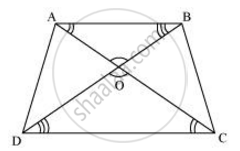# Diagonals AC and BD of a trapezium ABCD with AB || DC intersect each other at the point O. Using a similarity criterion for two triangles, show that (AO)/(OC) = (OB)/(OD) - Mathematics

Diagonals AC and BD of a trapezium ABCD with AB || DC intersect each other at the point O. Using a similarity criterion for two triangles, show that (AO)/(OC) = (OB)/(OD)

#### SolutionIn ΔDOC and ΔBOA,

∠CDO = ∠ABO [Alternate interior angles as AB || CD]

∠DCO = ∠BAO [Alternate interior angles as AB || CD]

∠DOC = ∠BOA [Vertically opposite angles]

∴ ΔDOC ∼ ΔBOA [AAA similarity criterion]

∴ (DO)/(BO) = (OC)/(OA)... [Corresponding sides are proportional]

⇒ (OA)/(OC) = (OB)/(OD)

Concept: Criteria for Similarity of Triangles
Is there an error in this question or solution?

#### APPEARS IN

NCERT Class 10 Maths
Chapter 6 Triangles
Exercise 6.3 | Q 3 | Page 139